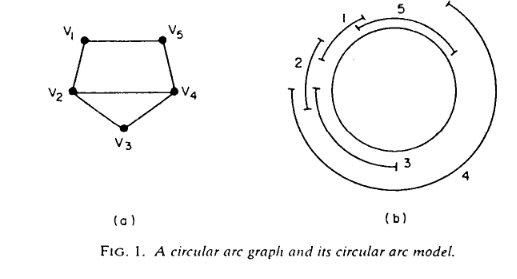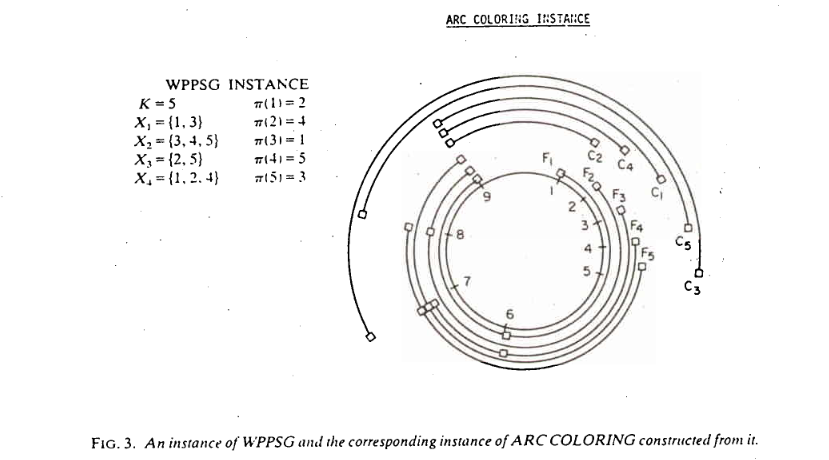# Tag Archives: WPPSG

## Arc Coloring

Last week we did a subproblem along the way to the next problem in the appendix.  This week we are making a second stop.

The problem: Arc Coloring.  This problem is not in the appendix.

The description: Given a circle divided into m equally spaced points, and a set {A1..An} of “arcs”.  Each arc Ai is represented by a pair (ai, bi) signifying an arc that starts at point ai and continues clockwise to point bi

A circular arc graph is a graph built from this set of arcs, with one vertex for each arc, and an edge between two arcs if they intersect at one or more points (the starting point of an interval ai does not count for overlap purposes)

The arc coloring question asks: Given an integer K, and a circular arc graph G, can we color G in K or fewer colors?  Alternately, we are asking whether we can partition the given set of arcs into K or fewer groups with no intersections within any group.

Example: The paper by Garey, Johnson, Miller, and Papadimitriou gives this example of a circular arc graph:The right side is the circle and the arcs, the left side is the graph.  So we can see there are edges connecting v1 to v2 and v5 because arc 1 overlaps with both arcs 2 and 5.

This graph can be colored with 3 colors (v2, v3, and v4 form a clique, so at least 3 colors will be needed, and coloring v1 the same color as v4 and v5 the same color of v1 will color the graph in just 3 colors).

Note that we already know that the general graph coloring problem is NP-Complete.  We are asking here whether this restricted kind of graph can be colored in polynomial time.  It will turn out that coloring this graph will be NP-Complete as well.

Reduction: The reason we talked about the Word Problem for Products of Symmetric Groups last time was to reduce from it here.

So we start with an instance of WPPSG- a collection of sets X1 through Xm, an integer K, and a goal permutation π.  We’ll assume that each number from 1 to K occurs in at least one X set (if not, if π moves that number we have an automatic “no” instance, and if it doesn’t, we can just remove the number from consideration (and shift all larger numbers down 1)).  We want to build a graph that is colorable in K colors or less if and only if our WPPSG instance is solvable.

Our circle will have K+m points on it.  Each set Xi will generate a set of arcs.  Let li be the index of the first X set that contains i, let li be the index of the second such set, and so on.  So using our example from last time, we’d say that l5 is 1 (because X1 is the first set to have a 5 in it), and l5 is 3 (because X3 is the second set to have a 5 in it).  We’ll denote the last such label as li[k(i)]

So, for each Xi we build a set of arcs:

• Ai1 = (i, K+li)
• Ai2 = (K+li, K+li)
• Ai,k(i) = (K+li[k(i)-1], K+li[k(i)])

This creates a set of arcs that are disjoint, and who cover the points from i to K+li[k(i)]

Each set Xi also creates an arc Ci = (K+li[k(i)], π-1(i)). The “π-1(i)” is just the inverse of the π permutation we started with, and tells us for each number from 1..K what position that number should end up in.  This Ci arc continues the Ai arcs from where they “end” and go around to π-1(i).

Here is an example of how this gets built from the paper:The actual proof that this conversion works is hard and complicated.  The general idea is that for each point in the circle, we need a different color for each arc that crosses the point.  The different colors can map to the permutations of the numbers from 1-K.  They show that you can build a permutation of colors that works based on the permutations you can do on the X sets, and so you can get to a legal permutation if and only if you can get to a legal coloring.

Difficulty: 8.  The construction is cool, but hard to see from the initial problem.  The actual proof is very tricky as well.

## Word Problem For Products of Symmetric Groups

The next problem in the Appendix is “Register Sufficiency for Loops”, which basically asks “Given a set of loop variables and K registers, can we assign variables to registers so that there is no conflict between loop iterations?”.  The reduction is better explained in two parts.  This is the first part.

The problem: The “Word Problem for Products of Symmetric Groups” (WPPSG).  This is not in the appendix.  It is defined in the paper by Garey, Johnson, Miller, and Papadimitriou that has the reduction we will want for the Register Sufficiency for Loops problem.

The description: Given an integer K, and m sets X1 through Xm, each containing a subset of {1..K}, and a permutation π of the numbers from 1-K. Can we get π by a series of permutations defined by permuting with X1, then X2, and so on through Xk?

Example:

To “permute with” an Xi set,  I mean we can rearrange the elements corresponding to the positions in Xi however we want, but elements that are not listed in Xi do not move.

So, suppose we had K=5 and 3 sets:

X1 = {1,3,5}

X2 = {2,3}

X3 = {4,5}

If π = {3,1,2,5,4}, we can do this by first permuting with Xusing {3.1,5} turning {1,2,3,4,5} into {3,2,1,4,5}.  Then we permute X2 using {3,2},turning {3,2,1,4,5} into {3,1,2,4,5}.  Finally, we permute X3, using {5,4} turning {3,2,1,4,5} into {3,2,1,5,4}.

If π = {2,1,5,3,4} we can’t do it.  There is no way to get a 2 into the first location, since the only permutation that can possibly place something in the first location is X1, and we have to do the X permutations in order.

Reduction:

If you’re following along, this is Theorem 2 of the paper.  They go from Disjoint Connecting Paths, but add the restriction that the graphs are directed acyclic graphs.  (They say it’s a “trivial modification” to a standard reduction.  We did that reduction slightly differently, but since we went from a flow problem, I think it is fine to convert that to do a DAG version of the problem reduction from a directed version of the flow problem).

They also modify the problem so that the start vertices all have indegree 0 (and all vertices with indegree 0 are start vertices), and all end vertices have outdegree 0 (and all vertices with outdegree 0 are end vertices).

So, we’re given a DAG G, and a set of start vertices s1..sn along with their matching end vertices t1..tn.  First, we will take the graph and replace all edges a= (u,v) with a pair of edges going through a new vertex just for edge a: (u, wa) and (wa, v).  That new vertex is “private” to the edge and is not used anywhere else.  Our new graph G’ has q vertices.

Since we do have a DAG, we can topologically sort the vertices.  Set up a sorting so that the first vertices in the sort are the vertices s1..sn. We can get away with this because all of the s vertices have indegree 0. Similarly, make the last vertices in the sorting be t1..tn.

For each vertex vi, define B(i) = {j:(vi, vj) is an edge}.

Now we’re ready to build our WPPSG instance.  Our X sets are:

Xj = {n+j} ∪ B(n+j) for j from 1 to q-n

The last set is Xq-n+1 = {1,2,…q-n}

The permutation we want to build is:

π(i) = q-n+i for i from 1 to n

π(i) = i-n for i from n+1 to q.

Our WPPSG instance has one position in its permutation for each vertex in G’.  Every position starts labeled by its own index.  Each set Xi contains vertex vn+1 and its immediate predecessors.  So permutation X1 will replace s1 with a label of one of its neighbors, which corresponds to moving along that edge.  Suppose we permute s1 with some vertex v.  Then when we get to Xv, we will permute with one of v’s neighbors, and so on down the chain until we get the final position on a t vertex.  The final permutation ensures that we have eventually permuted the labels to end up with their corresponding t vertices.  (It doesn’t matter what happens with the rest of the labels).

Difficulty: 7.  I like the idea of “pushing” a path through a graph into a permutation problem.